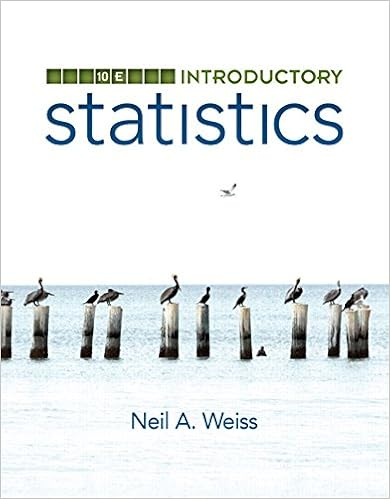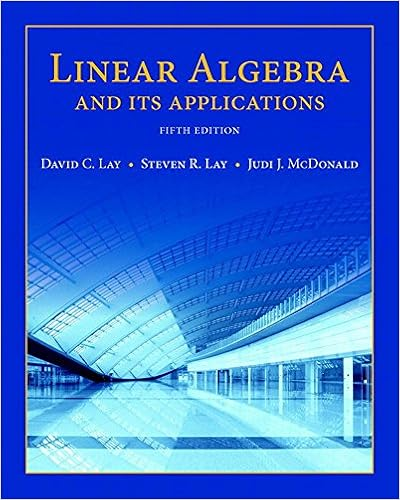# MATH 253 2010 Midterm 2 Version D Solutions -...

• Test Prep
• 5

This preview shows page 1 - 2 out of 5 pages.

The preview shows page 1 - 2 out of 5 pages.
Calculus II Midterm 2March, 201021. (3 marks each; total 12 marks)Answer each question in the space provided.YouMUST show your work.a)Given the parametric equationstx25+=,1962++=ttyfinddxdyevaluated at the point (7, 16).b)Consider a 60 L tank filled with water, initially containing 0.03 kg of salt per litre.Water containing 0.1 kg/L of salt flows into the tank at the rate of 5 L/min. If thesalt-water solution in the tank is thoroughly mixed and flows out from the tank ata rate of 5 L/min, then set upthe differential equation describing the rate ofchange of salt (call itA) in kg in the tank as a function of time (call itt) inminutes, and state the corresponding initial condition.You do NOT have to solvethe equation.c)Ifuvwwvwuwvuf53cos),,(42++=, findvd)For what nonzero values ofkdoes the functionktBktAycossin+=satisfythe differential equation04=+yy?State all possible values.f

Course Hero member to access this document

##### We have textbook solutions for you!The document you are viewing contains questions related to this textbook.
Chapter 8 / Exercise 23
Calculus
StewartExpert VerifiedBrowse all Textbook Solutions

Course Hero member to access this document

End of preview. Want to read all 5 pages?

Course Hero member to access this document

Term
Winter
Professor
staff
Tags
Math, Constant of integration, Boundary value problem
##### We have textbook solutions for you!
The document you are viewing contains questions related to this textbook.The document you are viewing contains questions related to this textbook.
Chapter 8 / Exercise 23
Calculus
StewartExpert Verified
•••